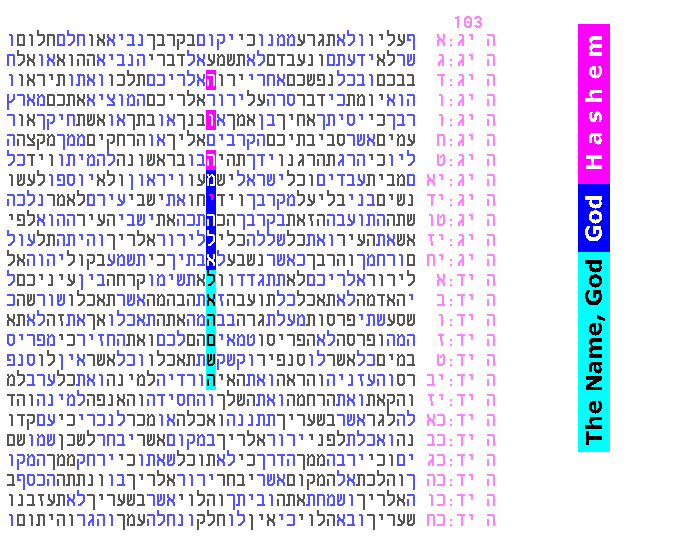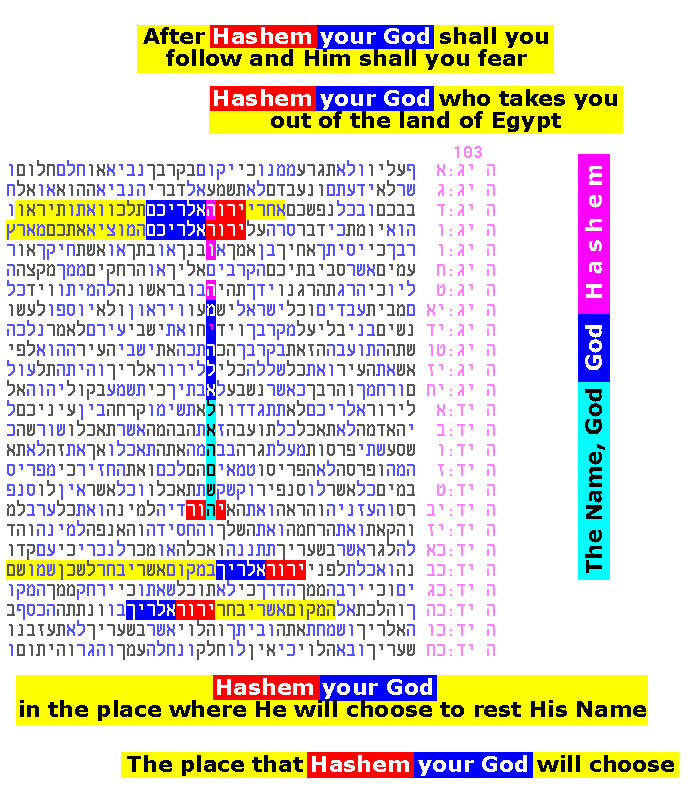Motivations/ Essential Evidence Evidence Newest Limitations Introduction as of 2007 as of 2010 EvidenceHOME   PARTICIPATEJuly 2010: The codes using the Names of G-d - as seen in the previous few pages (here and here) were already at the 1-in-a-million level. We have now found that those codes point to yet other codes, shown below. These newest discoveries also exceed the 1-in-a-million (1:1M) threshold, and they are some of the simplest for the layman to verify (see Figure 21a's explanation). A result of 1:1M on top of a previous result of 1:1M is not in any way "normal".

The new codes all arose from two observations from subscriber Tim Brooks. First, he saw the additional expression "The Name, G-d" (pronounced "Hashem, HaKal") when looking at existing Figure 16. This expression is shown below in light blue in Figure 20a. G-d is actually referred to as "The Name" (Hashem) in Deuteronomy 28:58, and He is referred to as "The G-d" (HaKal) in Deuteronomy 7:9, shown in this previous Figure.Figure 20a

This light blue ELS acts like a "code about a code" (i.e. it leads us to one or more other codes). The ELS includes "Kal", and that word leads us to another area in the same table, a cluster of 11 Kal occurrences (Figure 20b below). "Shakkai" is actually written in the Torah by itself as well as with the prefix "Kal" (in fact it appears as Kal Shakkai in the verse that launched all of these codes, Exodus 6:3). Therefore, the presence of Kal here is very appropriate.Figure 20b

Kal also appears in a cluster in our original table that launched the whole series:Figure 20c

Figure 20b and 20c contain the most significant Shakkai occurrences in our series, and we see that in both cases, the Kal prefix clusters strongly near these Shakkaiappearances, with an estimated combined significance beyond 1:1,000.

In addition, Tim Brooks observed that the entire new expression, "The Name, G-d" in Figure 20a leads us directly to the following code in Figure 21a. In Figure 20a, "The Name, G-d" is adjacent to the two Names Hashem and G-d (the latter being pronounced Elokim, in dark blue). These same two Names appear with the same starting expression "The Name, G-d" in Figure 21a:Figure 21a

Layman's verification:

Figure 21a is a same-column code, and its significance is higher than 1:100,000. This can be verified by a layman by doing a few multiplications, as follows. First, we note that in the same-column format, the position of Elokim (dark blue, translated in the figure as "G-d") relative to "The Name, G-d" is in the best position possible (the two ELSs form a single string, and both are in the same direction). Elokim contains 5 letters. Let's estimate conservatively and say that each of the 5 letters has 1 chance in 10 of appearing exactly where we see it (why this is conservative). The whole word Elokim appearing where it does therefore has chances of 1/10 multiplied by itself 5 times, for a total estimate of 1:100,000 for this meeting alone. This multiplication is legitimate only because the first code (Figure 20a) defined for us what to look for in the second code (Figure 21a), with very few alternatives.

We actually need to decrease the estimated significance due to these alternatives (for example, Hashem could have appeared as an extension, instead of Elokim). And we decrease the significance further because our starting ELS, "The Name, G-d" (at skip 103 in the table) is not the minimal skip ELS for this expression - there are 12 ELSs for "The Name, G-d" with skips smaller than 103. But these decreases are more than balanced by an increase in significance due to the presence of Hashem so close by in the same column.

In fact, computer runs give results in the same ballpark as our initial estimate. When we have the computer compare this Torah result to thousands of monkey texts using the same-column criterion, we find a more compact monkey text arrangement for the 3 expressions (The Name, G-d; Elokim; Hashem) only 4 times in 500,000 trials.

This result, combined with the Kal result, bring these new discoveries beyond the level of 1:1M. There are other possible ways of looking for Kal and for "The Name, G-d", which may or may not succeed, but what we have tried so far are among the most obvious ways of checking.

It is interesting to note the following additional phrases in Figure 21b below. These phrases appear in the plain text that surrounds the new code (we do not assign probabilities for such crossing phrases, but we do notice them):Figure 21b

Verses including the words "the place, Hashem/G-d/He, will choose, to rest, His Name", occur about 7 times in Torah. Three of these occur in our short series, one in the above table, and one each in previous pages' Figure 2b and Figure 17.